# Propositional Logic Statement Proposition A Statement is a

• Slides: 26Propositional LogicStatement (Proposition) A Statement is a sentence that is either True or False Examples: 2+2=4 True 3 x 3=8 False 787009911 is a prime Non-examples: x+y>0 x 2+y 2=z 2 They are true for some values of x and y but are false for some other values of x and y.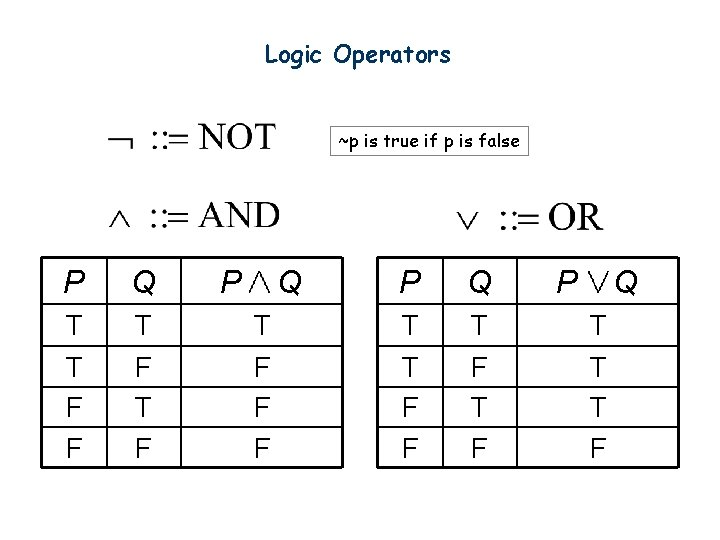Logic Operators ~p is true if p is false P Q T T F F T F P Q T F F F P Q T T F F T F P Q T T T F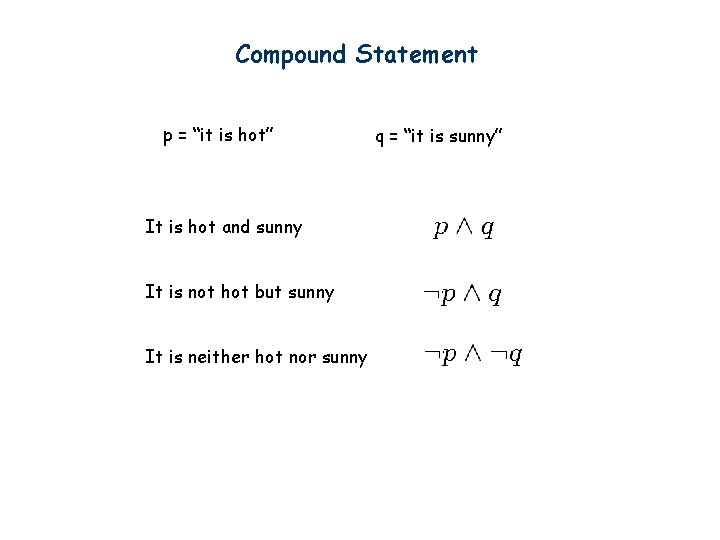Compound Statement p = “it is hot” It is hot and sunny It is not hot but sunny It is neither hot nor sunny q = “it is sunny”Exclusive-Or coffee “or” tea exclusive-or How to construct a compound statement for exclusive-or? p T T F F q T F p q F T T F Idea 1: Look at the true rows Want the formula to be true exactly when the input belongs to a “true” row. The input is the second row exactly if this sub-formula is satisfied And the formula is true exactly when the input is the second row or the third row.Exclusive-Or coffee “or” tea exclusive-or How to construct a compound statement for exclusive-or? p T T F F q T F p q F T T F Idea 2: Look at the false rows Want the formula to be true exactly when the input does not belong to a “false” row. The input is the first row exactly if this sub-formula is satisfied And the formula is true exactly when the input is not in the 1 st row and the 4 th row.Logical Equivalence Idea 3: Guess and check p q T T F F T T T T F F T F Logical equivalence: Two statements have the same truth table As you see, there are many different ways to write the same logical formula. One can always use a truth table to check whether two statements are equivalent.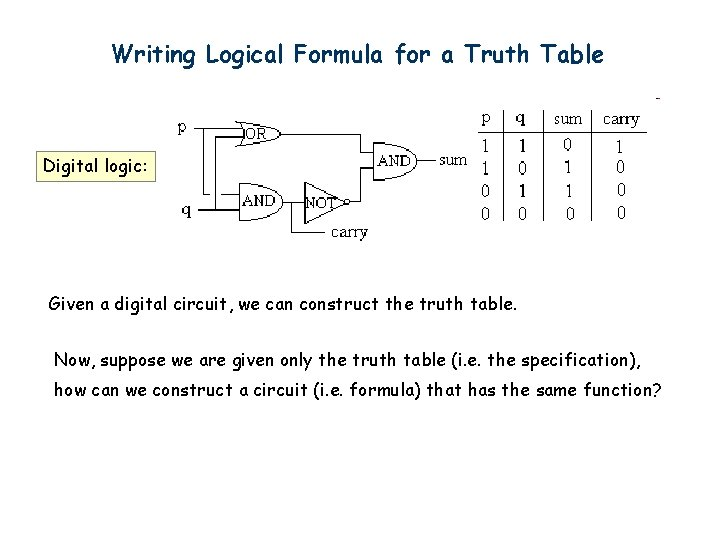Writing Logical Formula for a Truth Table Digital logic: Given a digital circuit, we can construct the truth table. Now, suppose we are given only the truth table (i. e. the specification), how can we construct a circuit (i. e. formula) that has the same function?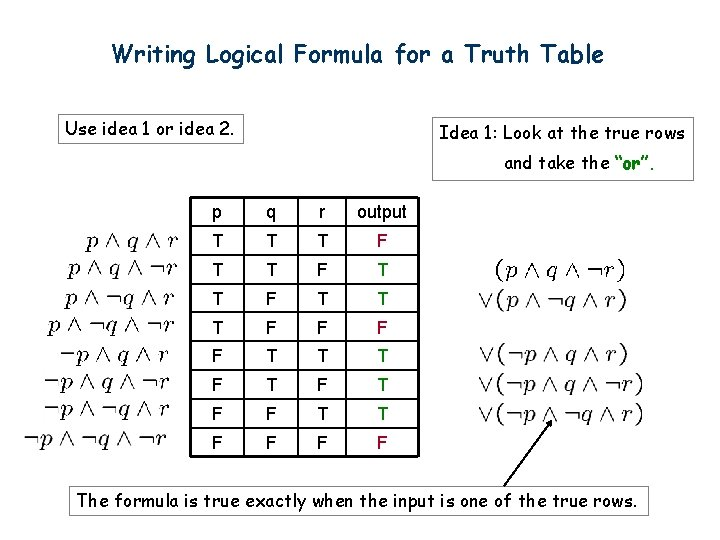Writing Logical Formula for a Truth Table Use idea 1 or idea 2. Idea 1: Look at the true rows and take the “or”. p q r output T T T F T T T F F T T T F T F F The formula is true exactly when the input is one of the true rows.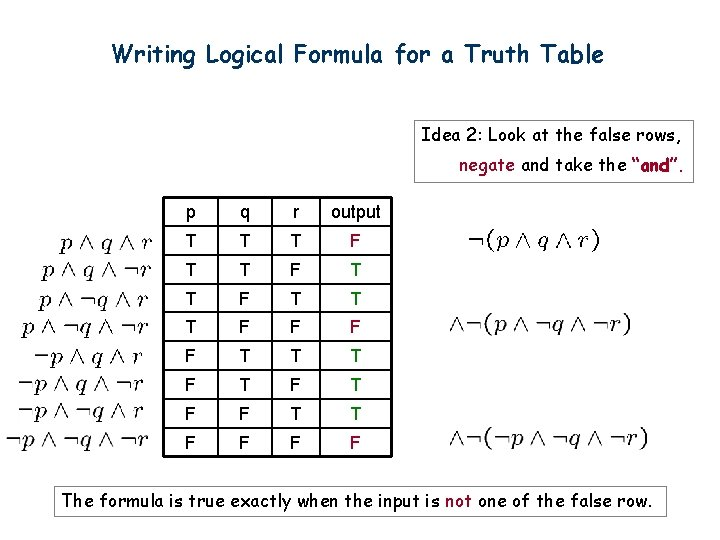Writing Logical Formula for a Truth Table Idea 2: Look at the false rows, negate and take the “and”. p q r output T T T F T T T F F T T T F T F F The formula is true exactly when the input is not one of the false row.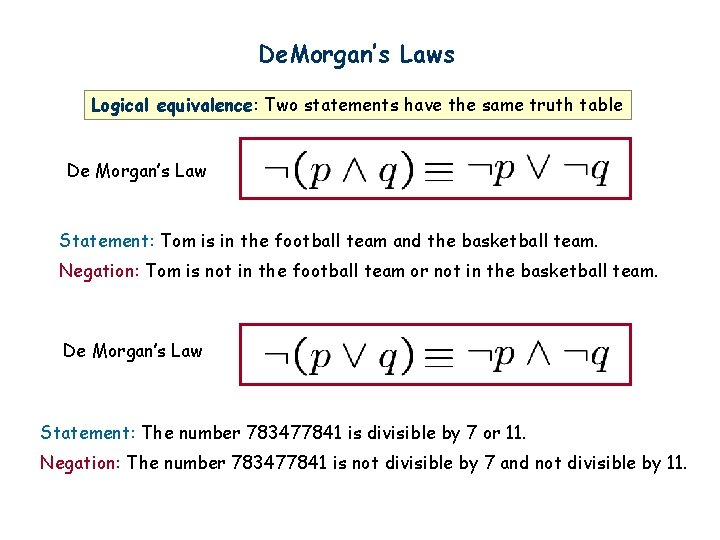De. Morgan’s Laws Logical equivalence: Two statements have the same truth table De Morgan’s Law Statement: Tom is in the football team and the basketball team. Negation: Tom is not in the football team or not in the basketball team. De Morgan’s Law Statement: The number 783477841 is divisible by 7 or 11. Negation: The number 783477841 is not divisible by 7 and not divisible by 11.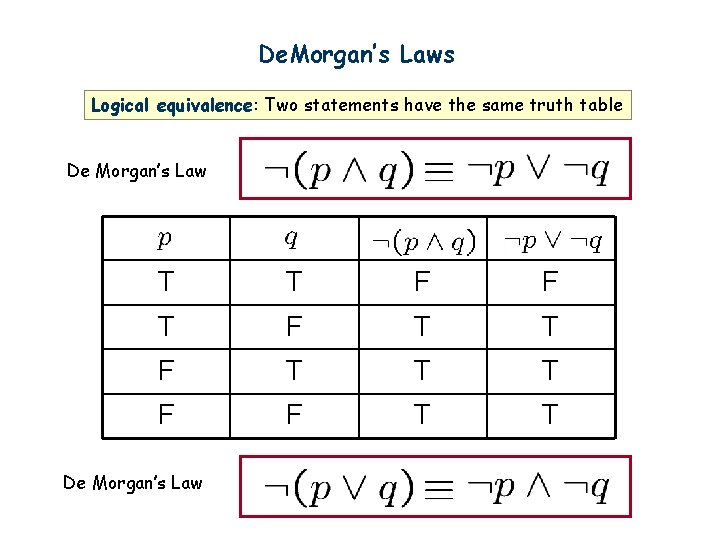De. Morgan’s Laws Logical equivalence: Two statements have the same truth table De Morgan’s Law T T F F T T T F F T T De Morgan’s Law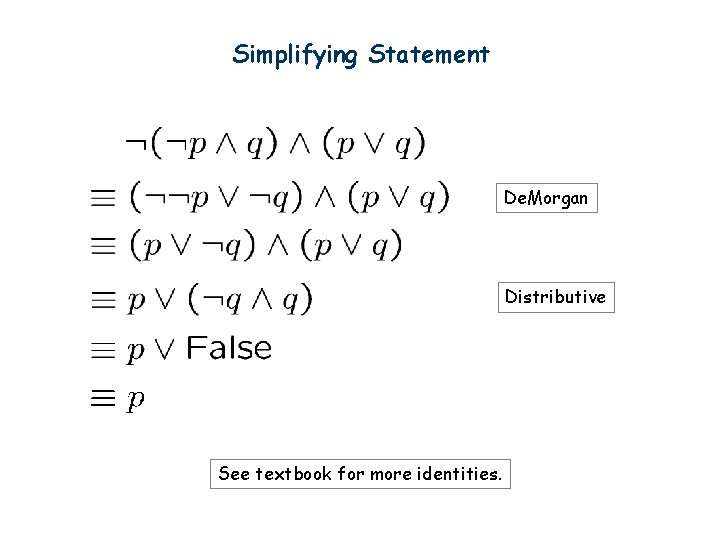Simplifying Statement De. Morgan Distributive See textbook for more identities.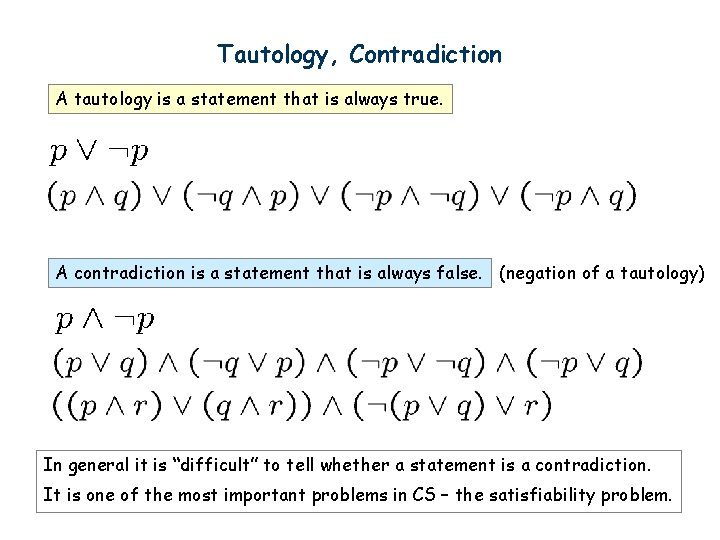Tautology, Contradiction A tautology is a statement that is always true. A contradiction is a statement that is always false. (negation of a tautology) In general it is “difficult” to tell whether a statement is a contradiction. It is one of the most important problems in CS – the satisfiability problem.Quick Summary Key points to know. 1. Write a logical formula from a truth table. 2. Check logical equivalence of two logical formulas. 3. De. Morgan’s rule and other simple logical rules (e. g. distributive). 4. Use simple logical rules to simplify a logical formula.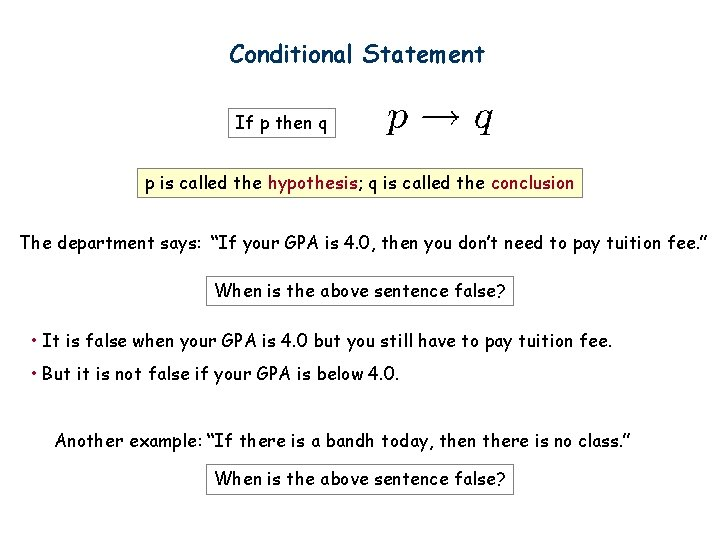Conditional Statement If p then q p is called the hypothesis; q is called the conclusion The department says: “If your GPA is 4. 0, then you don’t need to pay tuition fee. ” When is the above sentence false? • It is false when your GPA is 4. 0 but you still have to pay tuition fee. • But it is not false if your GPA is below 4. 0. Another example: “If there is a bandh today, then there is no class. ” When is the above sentence false?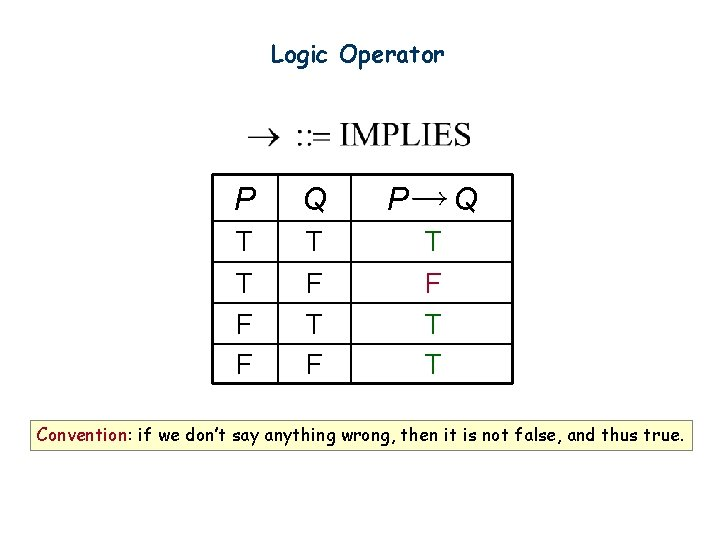Logic Operator P Q T T F F T F P Q T F T T Convention: if we don’t say anything wrong, then it is not false, and thus true.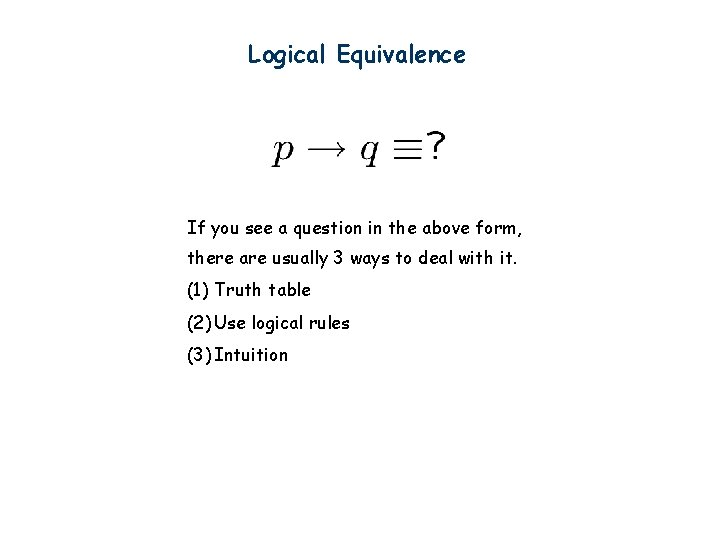Logical Equivalence If you see a question in the above form, there are usually 3 ways to deal with it. (1) Truth table (2) Use logical rules (3) Intuition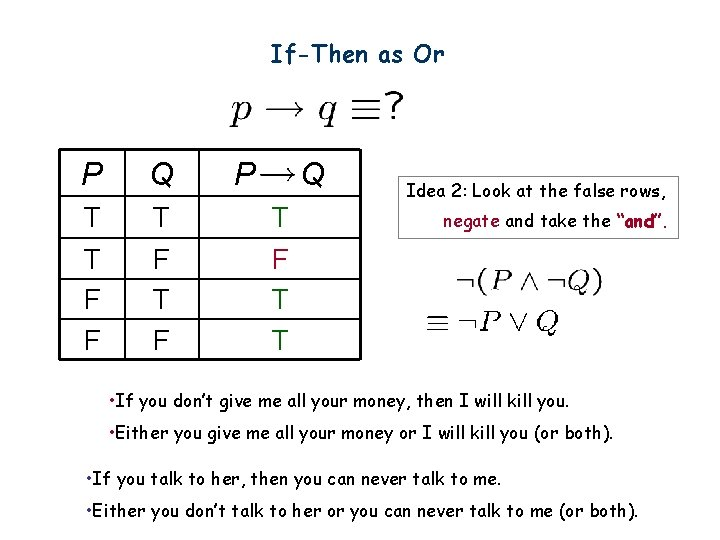If-Then as Or P Q T T F F T F P Q T F T T Idea 2: Look at the false rows, negate and take the “and”. • If you don’t give me all your money, then I will kill you. • Either you give me all your money or I will kill you (or both). • If you talk to her, then you can never talk to me. • Either you don’t talk to her or you can never talk to me (or both).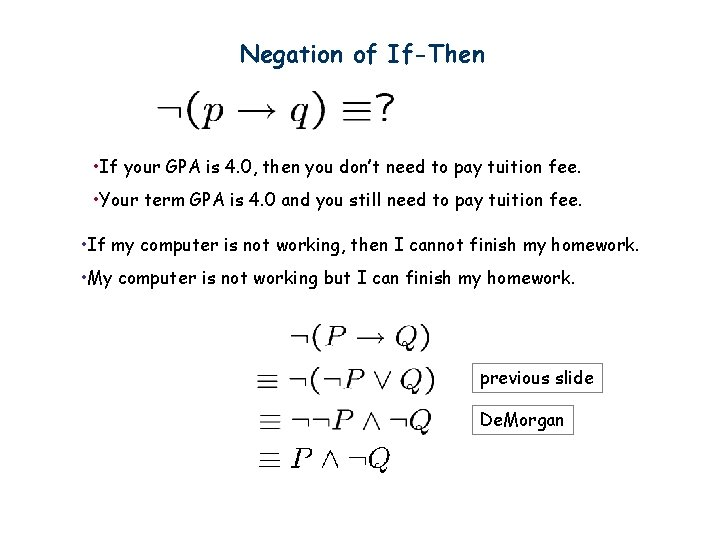Negation of If-Then • If your GPA is 4. 0, then you don’t need to pay tuition fee. • Your term GPA is 4. 0 and you still need to pay tuition fee. • If my computer is not working, then I cannot finish my homework. • My computer is not working but I can finish my homework. previous slide De. Morgan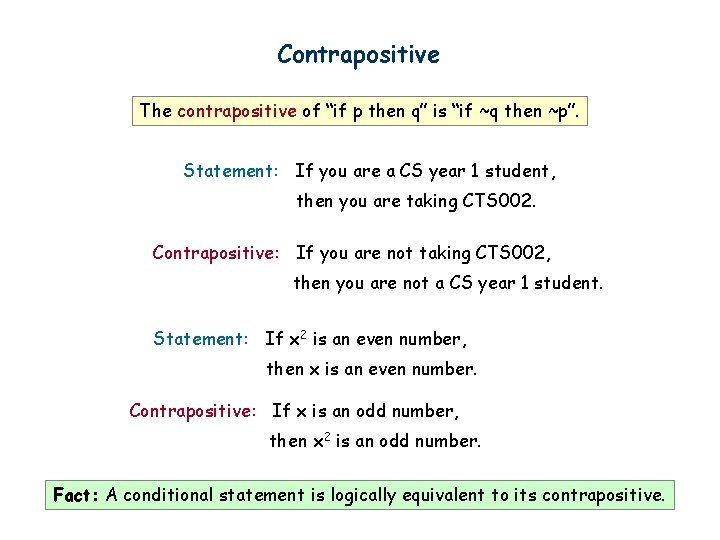Contrapositive The contrapositive of “if p then q” is “if ~q then ~p”. Statement: If you are a CS year 1 student, then you are taking CTS 002. Contrapositive: If you are not taking CTS 002, then you are not a CS year 1 student. Statement: If x 2 is an even number, then x is an even number. Contrapositive: If x is an odd number, then x 2 is an odd number. Fact: A conditional statement is logically equivalent to its contrapositive.Proofs Statement: If P, then Q Contrapositive: If Q, then P. T T T F F F T T F F T T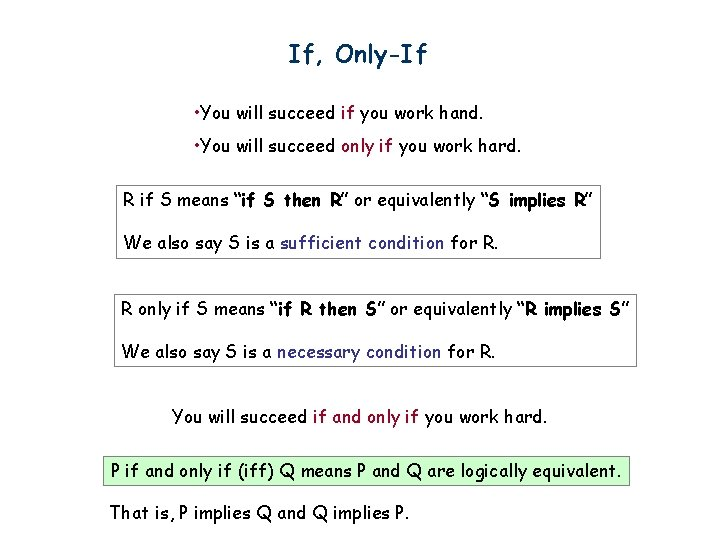If, Only-If • You will succeed if you work hand. • You will succeed only if you work hard. R if S means “if S then R” or equivalently “S implies R” We also say S is a sufficient condition for R. R only if S means “if R then S” or equivalently “R implies S” We also say S is a necessary condition for R. You will succeed if and only if you work hard. P if and only if (iff) Q means P and Q are logically equivalent. That is, P implies Q and Q implies P.Math vs English Parent: if you don’t clean your room, then you can’t watch a DVD. C This sentence says In real life it also means D So Mathematician: if a number x greater than 2 is not an odd number, then x is not a prime number. This sentence says But of course it doesn’t mean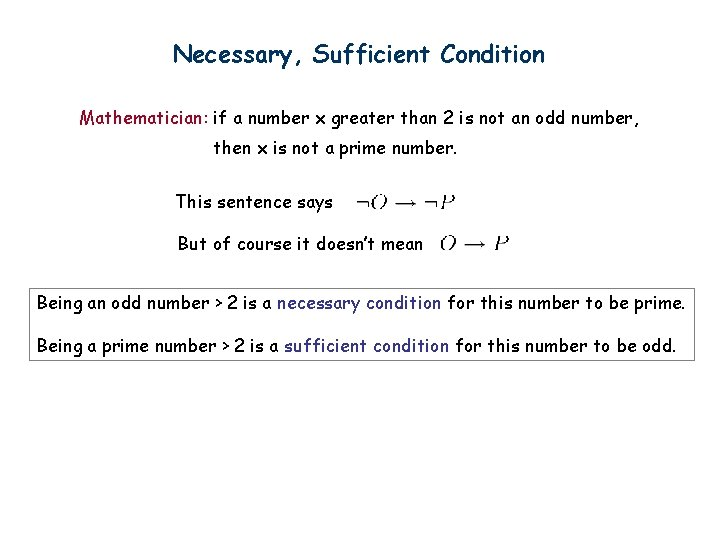Necessary, Sufficient Condition Mathematician: if a number x greater than 2 is not an odd number, then x is not a prime number. This sentence says But of course it doesn’t mean Being an odd number > 2 is a necessary condition for this number to be prime. Being a prime number > 2 is a sufficient condition for this number to be odd.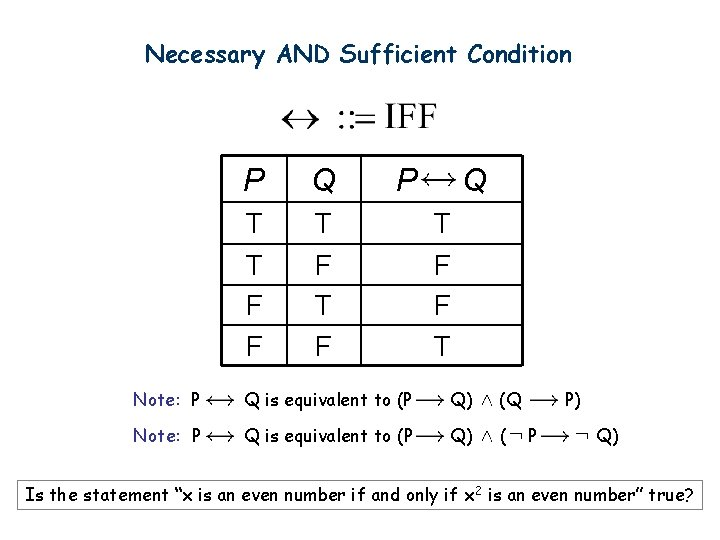Necessary AND Sufficient Condition P Q T T F F T F P Q T F F T Note: P Q is equivalent to (P Q) (Q Note: P Q is equivalent to (P Q) ( P) P Q) Is the statement “x is an even number if and only if x 2 is an even number” true?